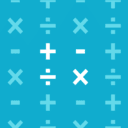# Differential Calculus (2017 edition)Start from the basics
Learn the basics, starting with Limits introduction
Let's go## Get StartedLimits introduction

A limit is the value that a function or sequence "approaches" as the input or index approaches some value. In this tutorial, we supply an intuitive understanding of limits.
Start learning